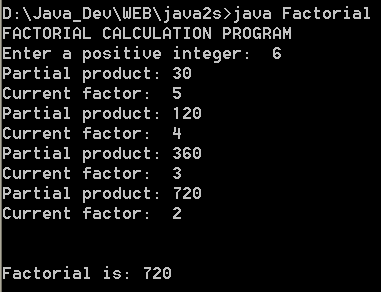# Use toString method of Long class to convert Long into String. : long « Data Type « Java

Use toString method of Long class to convert Long into String.

```

public class Main {

public static void main(String[] args) {

Long lObj = new Long(10);

String str = lObj.toString();

System.out.println("Long converted to String as " + str);

}

}

```

### Related examples in the same category

 1 Long class creates primitives that wrap themselves around data items of the long data type 2 Calculate factorial of integers up to this value3 Min and Max values of datatype long 4 Java long Example: long is 64 bit signed type 5 Convert Java String to Long example 6 Convert long primitive to Long object Example 7 Convert Long to numeric primitive data types example 8 Create a Long object 9 Convert String to long Example 10 Compare Two Java long Arrays Example 11 Java Sort long Array Example 12 Convert bytes to megabytes 13 Converting a String to a long type Number 14 Convert from String to long 15 Convert from long to String 16 Compute prime numbers 17 Returns the sign for long value x 18 Gets the maximum of three long values. 19 Gets the minimum of three long values. 20 A utility class for converting a long into a human readable string. 21 Add two long integers, checking for overflow. 22 Int To Long Compressor